## 3.2 Chaos Game

The method of generating fractals presented in the previous chapter is based on recursion. By composing the transformations, the number of objects we need to draw grows exponentially. In the case of the fern, which consists of four transformations, generating a fern at a depth of 10 requires drawing $$4^{10}$$ points (more than a million). And 10 levels is very little if we want to get attractive high-resolution graphics. If we wanted to perform $$56$$ iterations for a Sierpiński carpet, we would have to draw $$8^{56}>10^{50}$$ points, which is more than there are atoms on the whole Earth!

It turns out that fractals based on the composition of contractions can be generated in a more controlled way. Moreover, it will turn out to be based on the generation of random movements.

Readers, it will be amazing! Performing random movements repeatedly will continuously lead us to invariably constant geometric figures. It turns out that even long random trajectories can lead to predictable patterns, and this will be the chaos game.

### 3.2.1 Chaos game and triangles

Let’s start with a simple example, which will be a generalized method for constructing the Sierpiński triangle. Let’s proceed with the following algorithm…

1. Choose any three non-collinear points on the plane. These will be the vertices $$A$$, $$B$$ and $$C$$ of the Sierpiński triangle.
2. Choose any point on the plane. This will be the position of our fractal drawing pen.
3. Pick a vertex $$A$$, $$B$$ or $$C$$ at random and move the pen by half in the direction of the selected vertex. Repeat this drawing and shifting indefinitely (or long enough).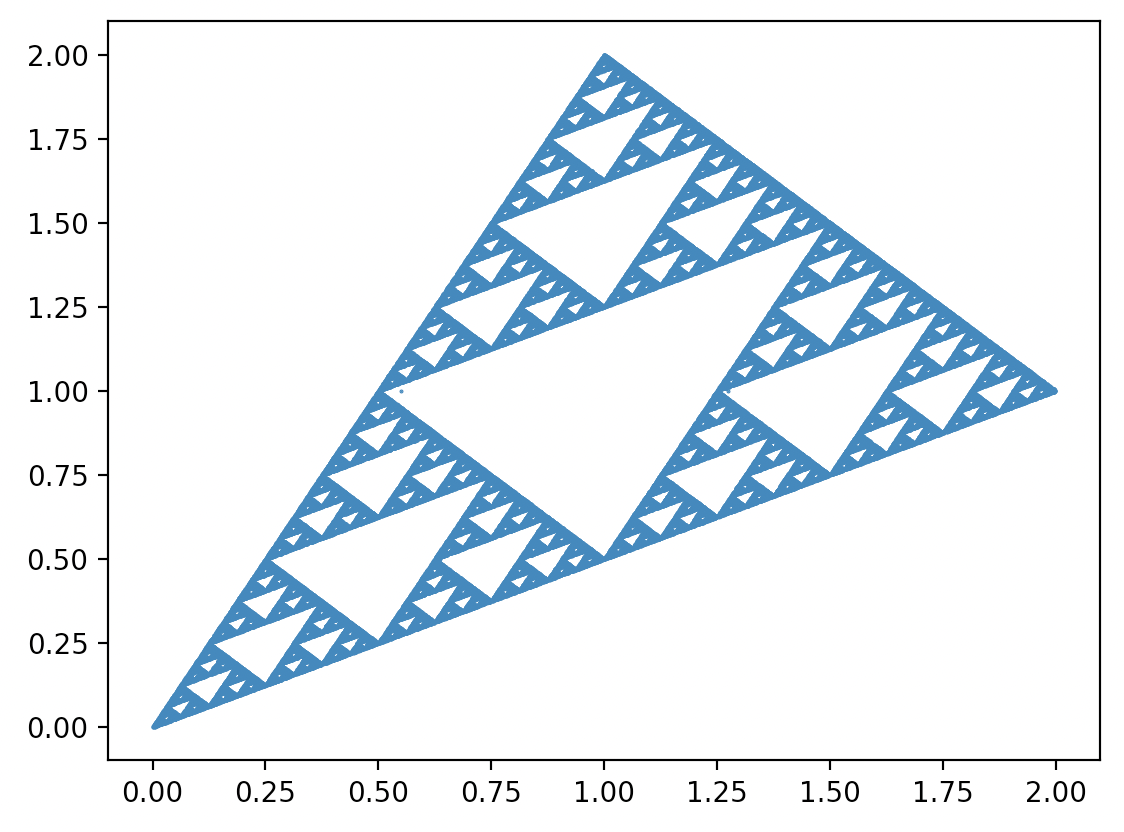Triangle composed of 100 000 points in the game of chaos

It turns out that when we follow the position of the pen, the Sierpiński triangle appears to our eyes!

### 3.2.2 What’s going on here?

Note, Dear Reader, that moving the pen by half the distance towards the selected vertex is equivalent to performing a transformation -> reduce the image by 1/2 and move towards the selected vertex.

Thus, in reality, we randomly perform one of the transformations: (1) reduce 2 times and move toward vertex A, (2) reduce 2 times and move toward vertex B, or (3) reduce 2 times and move toward vertex C.

In the previous chapter, we already used such transformations to build the Sierpiński triangle. But we used all three simultaneously, composing such a sum of transformations many times.

It turns out that we don’t have to perform these transformations at the same time, just draw one at a time and repeat this process.

### 3.2.3 Chaos game and fractals

The algorithm for generating fractals using the chaos game involves transforming a single point (pen) iteratively by a randomly selected transformation from a certain set of transformations. Each transformation must be a contraction (in the examples we will limit ourselves to affine transformations).

Algorithm for the construction of fractals based on the chaos game.

1. Choose a starting point for the pen.
2. From a set of contractions, choose one transformation and transform the pen coordinate according to this transformation.
3. Draw the pen coordinate on the screen.
4. Go back to step 2.

We will show how this algorithm works using Barnsley’s fern structure as an example, but for convenience we will first present an alternative way of writing affine transformations…

### 3.2.4 Affine transformations

In the previous chapter, we defined affine transformations by any combination of three types of transformations - translation, rotation and scaling (including vertical or horizontal reflection), with scaling having to have a scale of less than 1 in absolute value to be a contraction. Such a representation is convenient for people, because it is easy to imagine what happens to the output figures based on it. However, it turns out that for a computer a much more convenient representation of an affine transformation is the matrix form of the transformation.

In matrix notation, any affine transformation $$(x', y') = f(x, y)$$ in two-dimensional space can be represented by six numbers $$(a, b, c, d, e, f)$$.

$\begin{bmatrix} x' \\ y' \end{bmatrix} = \begin{bmatrix} a & b \\ d & e \end{bmatrix} \begin{bmatrix} x \\ y \end{bmatrix} + \begin{bmatrix} c \\ f \end{bmatrix} = \begin{bmatrix} a x + b y + c \\ d x + e y + f \end{bmatrix}.$

If we know the basics of matrix algebra, we can easily calculate the coefficients from the representation based on rotations, shifts and scaling $$a$$$$f$$. Some examples of corresponding representations of affine transformations are given in the next section.

But now let’s see how to construct a fern using the chaos game.

### 3.2.5 Ferns and chaos

Table 1 shows four affine transformations written using matrix notation.

a b c d e f
$$T_1$$: stem 0.00 0.00 0 0.00 0.16 0.00
$$T_2$$: top 0.85 0.04 0 $-$0.04 0.85 1.60
$$T_3$$: left leaf 0.20 $-$0.26 0 0.23 0.22 0.80
$$T_4$$: right leaf $-$0.15 0.28 0 0.26 0.24 0.44

[Coefficients of the transformation comprising Barnsley ferns]

To draw a fern from the figure below, just take a random point and repeat three steps 100,000 times: draw one of the transformations from this table, using draw probabilities of 1%, 79%, 10%, 10% for successive transformations, transform the coordinates of the point with the drawn transformation, draw the point on the graph.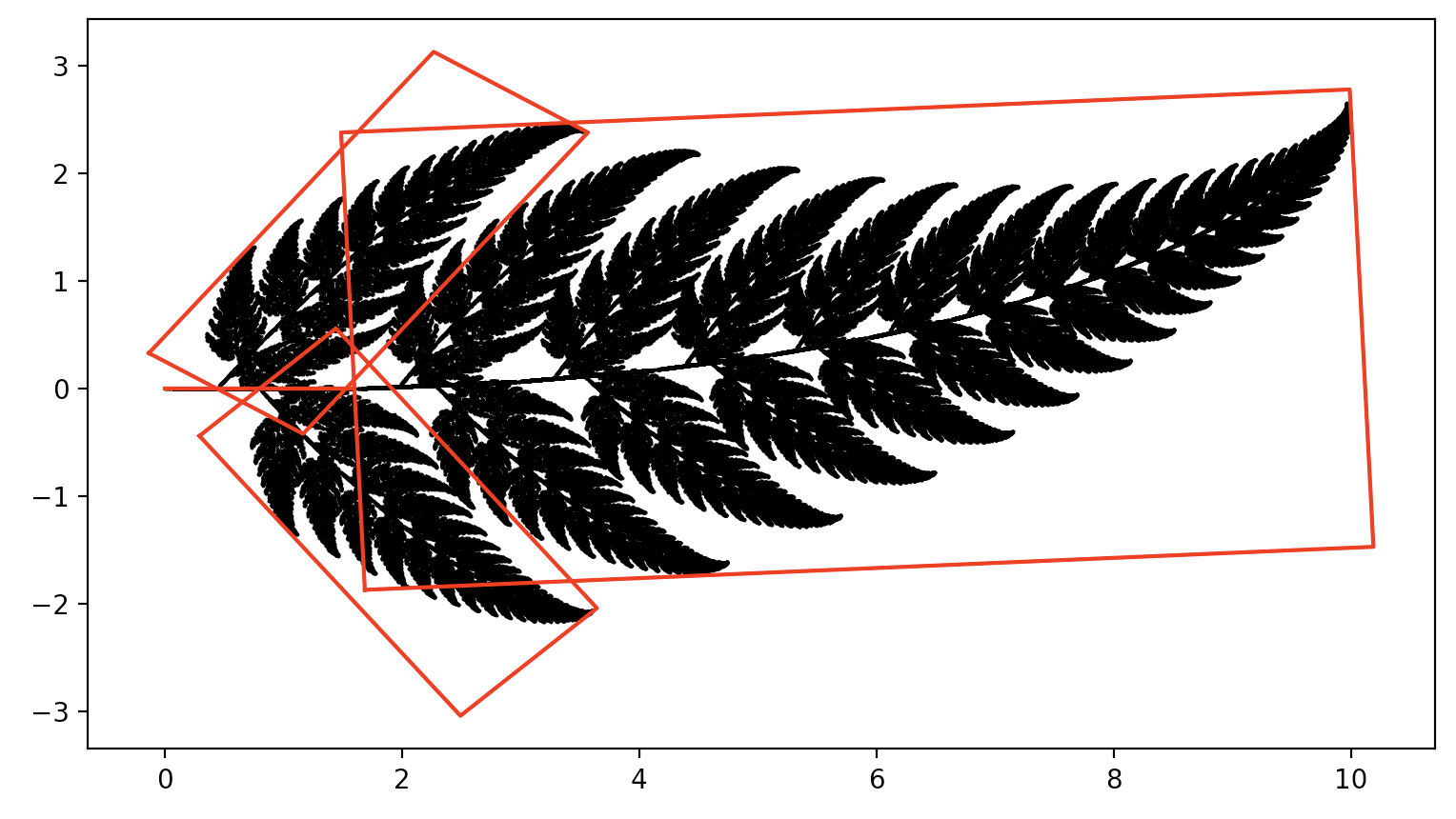Illustration of four transformations (outlined with red rectangles) based on Barnsley ferns

By the way, you can see that we have a huge reduction in information - such a beautiful fern was described using only 24 numbers. This observation became the foundation of fractal compression, a field that intended to significantly reduce the description of graphical figures using their fractal representation.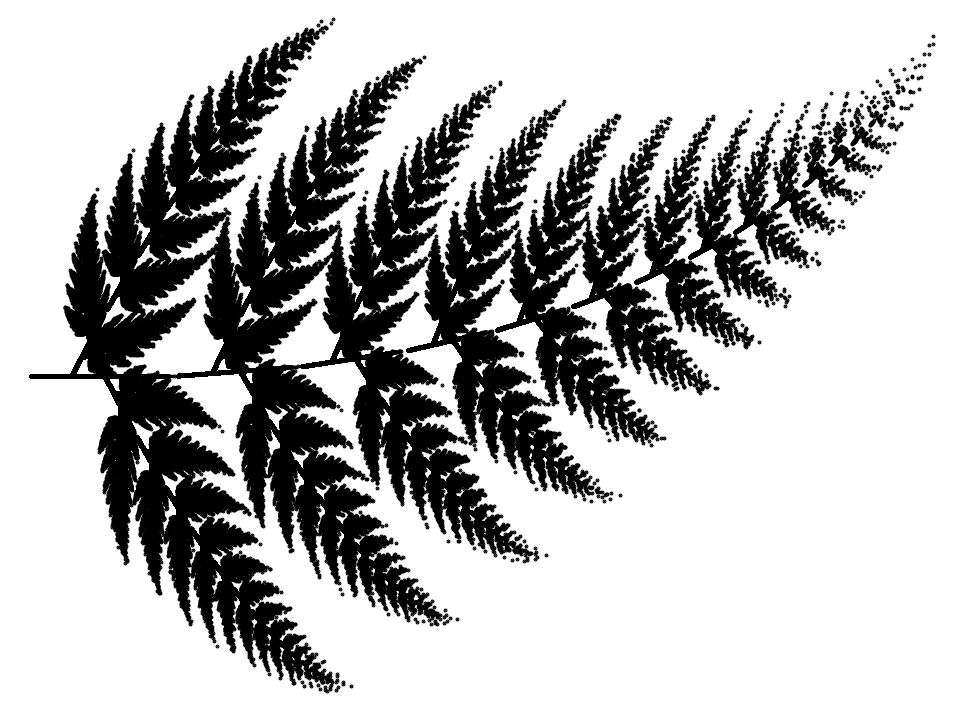More often we draw the left and right leaves, less often the top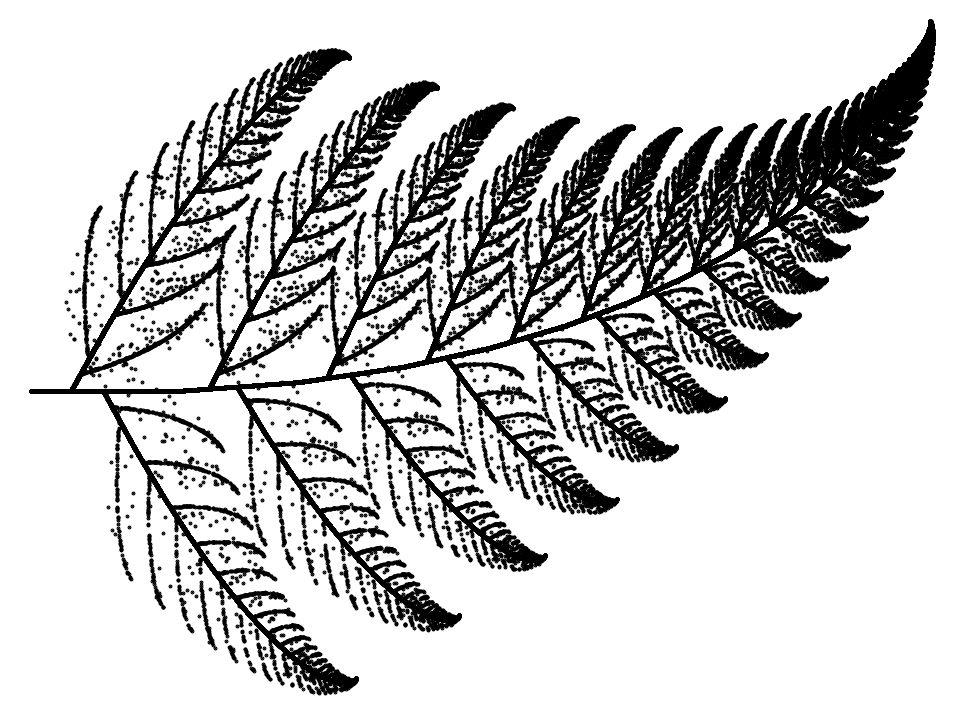More often we draw the stem, much less often the leaves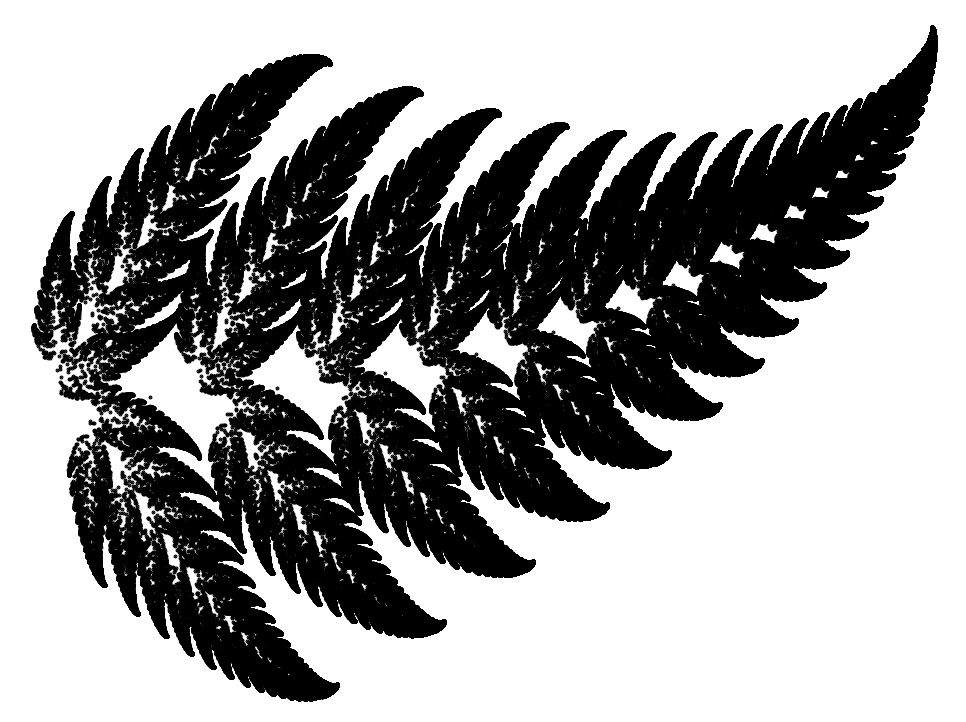We do not draw the stem

The choice of point $$x_0$$ doesn’t matter, but if it diverges a lot from the fractal, it’s worth skipping the first few steps of the chaos game for visual reasons, so that no points diverge too much from the main picture.

What role do probabilities play in the creation of a fractal?

Note that the probability of drawing the stalk is lower than the main part of the fern, also because the stalk takes up little space and there is no reason to waste time on drawing points from the stalk.

As an aside, we can look at three different Barnsley ferns that consist of the same transformations shown in Table 1, but in which different probabilities are used. The general outline of each of these ferns is similar, but we can see that by controlling the probabilities, we can get more or less perforated structures.

### 3.2.6 More formally

If we already have an intuition built up about how the game of chaos works, it’s a good idea to write down what happened more formally.

We have a set $$K$$ of transformations $$f_i:R^2 \rightarrow R^2$$, which are contractions, that is, they satisfy the condition:

$\exists_{\lambda < 1}\forall_{x, y} \lambda d(x,y) \geq d(f(x), f(y)).$

We have a vector K of probabilities of drawing consecutive transformations $$\pi_i \geq 0$$. The probabilities add up to 1, that is $$\sum_{i=1}^K \pi_i = 1$$.

A sequence of points $$x_0$$, $$f_{a_1}(x_0)$$, $$f^2(x_0) = f_{a_2}(f_{a_1}(x_0))$$, $$f^3(x_0) = f_{a_3}(f_{a_2}(f_{a_1}(x_0)))$$, … forms the image of a fractal. The symbol $$a_i$$ denotes the i-th drawn value from the set $$1...K$$ when drawing with probability $$\pi_1, ..., \pi_K$$.

The choice of point $$x_0$$ does not matter.